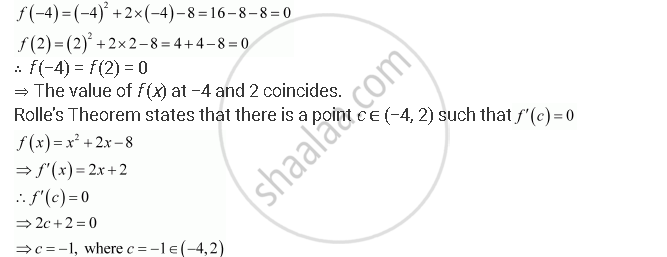Share

# Verify Rolle’S Theorem for the Function F (X) = X2 + 2x – 8, X ∈ [– 4, 2]. - CBSE (Science) Class 12 - Mathematics

#### Question

Verify Rolle’s theorem for the function f (x) = x2 + 2x – 8, x ∈ [– 4, 2].

#### Solution

The given function,f (x) = x2 + 2x – 8, being a polynomial function, is continuous in [−4, 2] and is differentiable in (−4, 2).Hence, Rolle’s Theorem is verified for the given function.

Is there an error in this question or solution?

#### APPEARS IN

NCERT Solution for Mathematics Textbook for Class 12 (2018 to Current)
Chapter 5: Continuity and Differentiability
Q: 1 | Page no. 186

#### Video TutorialsVIEW ALL 

Solution Verify Rolle’S Theorem for the Function F (X) = X2 + 2x – 8, X ∈ [– 4, 2]. Concept: Mean Value Theorem.
S